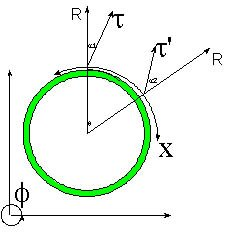# Fields of Dreams...Fields of Dreams...:)

I still remember the feeling of extreme perplexity when I was first introduced to the concept of Field.

It was either the concept of Gravitational or Electrostatic Field, and it happened sometime in my early childhood.

The concept of Field goes one step beyond the concept of Force at Distance.

A field is supposed to be a property of the space where a charge or a mass will sense a force and it is given by:

E =e/(4*pi*epsilon*r^2) for an Electric field

E =GM/r^2 for a Gravitational Field

It was mysterious for many reasons but I can tell you two. The first one is that somehow a charge or mass would modify space itself...:)

Einstein later linked Gravitation with modulations of the metric of a Riemannian spacetime continuum. The obvious inference that nobody draws for some obscure reason is that being the Electric Field 10^36 times greater than the Gravitational Field, one would expect that a single charge would produce a Black Hole.. :) and of course, light would bend around any capacitor... Of course, this is not the case. If you read the paper I, the HyperGeometrical Universe GUT, you know my solution to this problem.

The second amazing and very subtle property of fields is Extensivity, that is, fields are proportional to the number of particles (charges or mass) in a given region of space. You know, if you pick two one-pound bags of Coffee and put them together they weight two pounds.

Why is Extensivity such a mysterious property?

The reason is obvious. A geometrical theory of the Universe can only be made of metric oscillations (dilatons) and metric oscillators (dilators).

An important observation is that dilatons can carry angular momentum, that is, one can have a 4-D wave that will impact you with a 4-D torque. Just a reminder, spin has been associated in this theory with rotations perpendicular to R (4-Dimensional radial expansion direction) and one of the 3 spatial dimensions.

If Gravitation is an extensive property of matter, it means that all the dilators in a body are in phase. Had Newton or Gauss or anyone else tried to create a truly geometrical theory of the Universe, they would have to have reached the same conclusion I reached: There is a need for a Cosmological Coherence among Dilators and for a Coherence Lagrangian Principle governing Dilator Dynamics.

Why nobody asked "How come the things that generate the field are in phase? "

Newton, Gauss, Einstein and many others are the luminaries that helped Mankind to find their path to this point. Reverence, Respect, Admiration are appropriate emotions when considering their work. On the other hand, one has to question assumptions to evolve knowledge. I am doing my part in the questioning and do it with the highest admiration to my predecessors and stemmed colleagues.

Currently unrated

### New Comment

required

required (not published)

optional

required